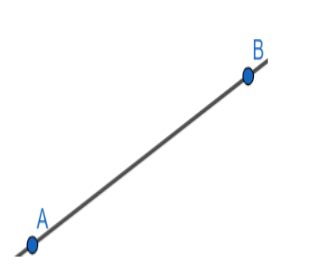Courses
Courses for Kids
Free study material
Free LIVE classes
MoreLIVE
Join Vedantu’s FREE Mastercalss

# If the points (p,0) , (0,q) and (1,1) are collinear , then $\dfrac{1}{p}+\dfrac{1}{q}$ is equal to:\begin{align} & \left( a \right) -1 \\ & \left( b \right) 1 \\ & \left( c \right) 2 \\ & \left( d \right) 0 \\ \end{align}Verified
363.6k+ views
Hint: We can approach this question by first writing the equation of the straight line that passes through two points by using the two point formula and then substituting the other point in the line equation in order to get the relation given in the question.

Firstly, points which lie on the same straight line are called collinear points.
Now in order to check the collinearity we need to first find the equation of the straight line that passes through the two given points.
The equation of straight line passing through two points can be found by using the two point formula.Let
\begin{align} & A\left( {{x}_{1}},{{y}_{1}} \right) \\ & B\left( {{x}_{2}},{{y}_{2}} \right) \\ \end{align}
Now from two point formula equation of straight line passing through A and B is
$\frac{x-{{x}_{1}}}{{{x}_{2}}-{{x}_{1}}}=\frac{y-{{y}_{1}}}{{{y}_{2}}-{{y}_{1}}}............\left( 1 \right)$
Given points are (p,0), (0,q) now on comparing these with the points $A\left( {{x}_{1}},{{y}_{1}} \right)$and $B\left( {{x}_{2}},{{y}_{2}} \right)$.
We get:
\begin{align} & {{x}_{1}}=p,{{y}_{1}}=0 \\ & {{x}_{2}}=0,{{y}_{2}}=q \\ \end{align}
Now on substituting these values in the two point formula from the equation (1) we get,
\begin{align} & \Rightarrow \dfrac{x-{{x}_{1}}}{{{x}_{2}}-{{x}_{1}}}=\dfrac{y-{{y}_{1}}}{{{y}_{2}}-{{y}_{1}}} \\ & \Rightarrow \dfrac{x-p}{0-p}=\dfrac{y-0}{q-0} \\ \end{align}
Now by simplifying it we get,
$\Rightarrow \dfrac{x-p}{-p}=\dfrac{y}{q}$
Now by simplifying the terms on both the sides we can write it as:
$\Rightarrow \dfrac{-x}{p}+1=\dfrac{y}{q}$
Then bring the x and y terms to one side and keep constant terms on the other side we get,
$\Rightarrow \dfrac{x}{p}+\dfrac{y}{q}=1................\left( 2 \right)$
Hence, the equation of line passing through (p,0) and (0,q) is $\dfrac{x}{p}+\dfrac{y}{q}=1$.
Now as (p,0) and (0,q) and (1,1) are collinear that means they lie on the same straight line which implies that the point (1,1) lies on the line $\dfrac{x}{p}+\dfrac{y}{q}=1.$
A point is said to lie on the line when it satisfies the equation of the given line.
So, now by substituting (1,1) in the equation $\dfrac{x}{p}+\dfrac{y}{q}=1.$we get,
$\therefore \dfrac{1}{p}+\dfrac{1}{q}=1$
Hence option (b) is correct.

Note- The equation of the straight line can also be found by using the slope point method but here it is ideal to use the two point method because in the question they already gave all the points required to apply that formula. However, both the methods will give the same result.
While writing the equation of the line if we cross multiply it, it will be little confusing to reach to the conclusion of $\dfrac{1}{p}+\dfrac{1}{q}$. So, it is better to simplify by dividing it with p on the left hand side there itself.
Last updated date: 30th Sep 2023
Total views: 363.6k
Views today: 9.63k